# Q&A: How to Look Up the Meaning of an Unknown Math Symbol or Expression

Cross-posted from here.

Here’s a general workflow for identifying the meaning of an unknown mathematical symbol/expression:

1. use an online tool (like webdemo.myscript.com/views/math) to convert a drawing of the symbol/expression into Latex commands
2. ask ChatGPT (chat.openai.com, free version 3.5 is fine) what the math symbol/expression given by those Latex commands means
3. based on ChatGPT's explanation, perform Google searches for more specific information/examples/fact-checking as needed

Here’s an demonstration on the expression $\displaystyle \sum_{i=1}^n \textrm E[X_i],$ which seems highly relevant to your specific use-case.

Step 1: Drawing $\to$ Latex Commands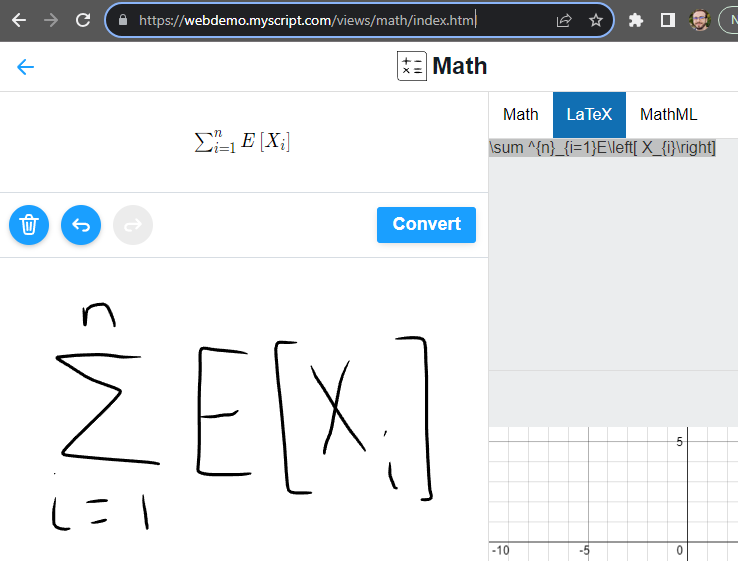Step 2: Latex Commands $\to$ Summary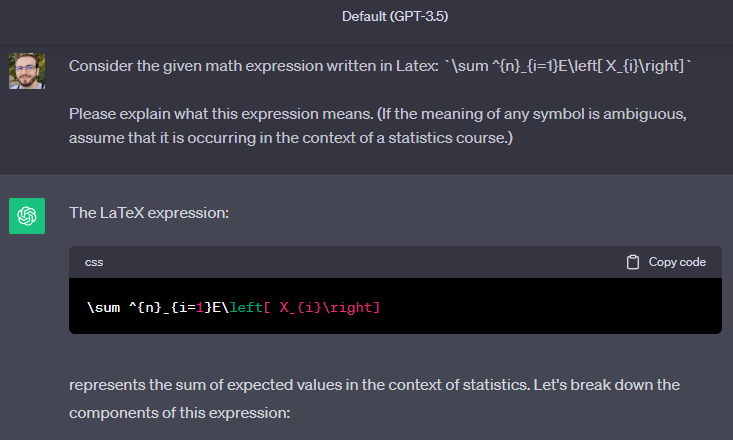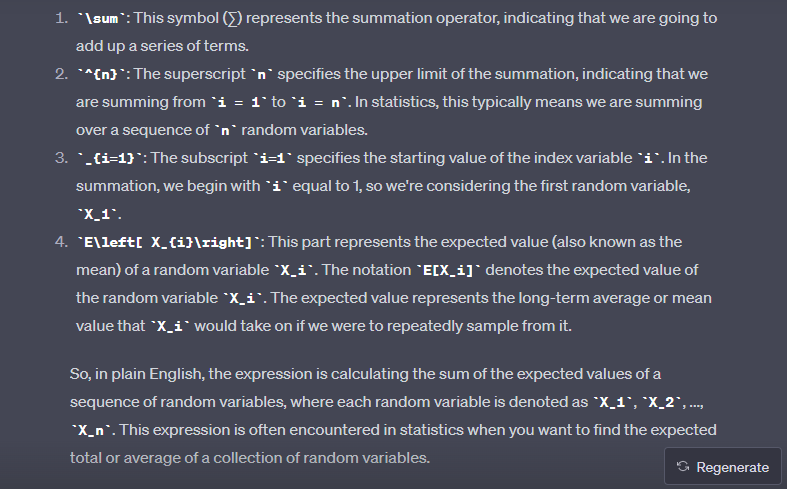Step 3: Summary $\to$ More Specific Information / Examples / Fact-Checking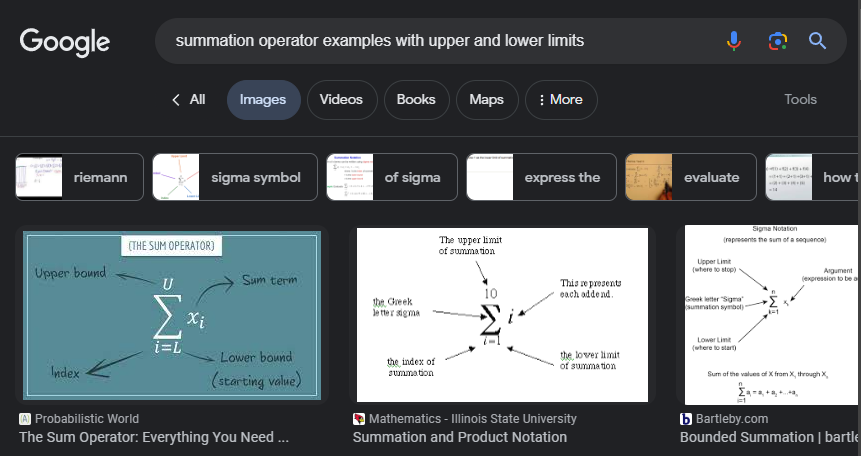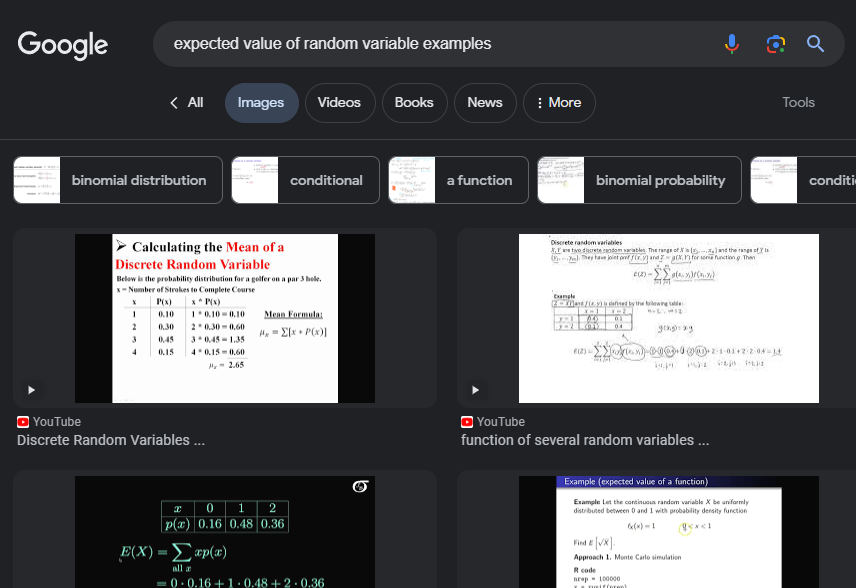Tags: